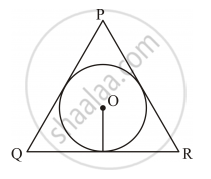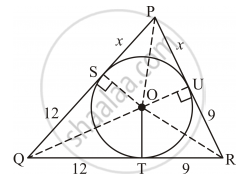Advertisement Remove all ads

# In Figure 5, a Triangle Pqr is Drawn to Circumscribe a Circle of Radius 6 Cm Such that the Segments Qt and Tr into Which Qr is Divided by the Point of Contact - Mathematics

Advertisement Remove all ads
Advertisement Remove all ads
Advertisement Remove all ads

In Figure 5, a triangle PQR is drawn to circumscribe a circle of radius 6 cm such that the segments QT and TR into which QR is divided by the point of contact T, are of lengths 12 cm and 9 cm respectively. If the area of ΔPQR = 189 cm2, then find the lengths of sides PQ and PR.Advertisement Remove all ads

#### Solution

Let PQ and PR touch the circle at points S and U respectively. Join O with P, Q, R, S and U.We have, OS = OT = OU = 6 cm (Radii of the circle)

QT = 12 cm and TR = 9 cm

∴ QR = QT + TR = 12 cm + 9 cm = 21 cm

Now, QT = QS = 12 cm (Tangents from the same point)

TR = RU = 9 cm

Let PS = PU = x cm

Then, PQ = PS + SQ = (12 + x) cm and PR = PU + RU = (9 + x) cm

It is clear that

ar (ΔOQR) + ar (ΔOPR) + ar (ΔOPQ) = ar (ΔPQR)

rArr 1/2xxQRxxOT+1/2xxPRxxOU+1/2xxPQxxOS=189     (given : ar(Δ PQR)=189cm^2)

rArr 1/2xx21xx6+1/2xx(9+x)xx6+1/2xx(12+x)xx6=189

rArr 1/2xx6(21+9+x+12+x)=189

⇒ 3 (42 + 2x) = 189

⇒ 42 + 2x = 63

⇒ 2x = 21

⇒ x = 10.5

Thus, PQ = (12 + 10.5) cm = 22.5 cm and PR = (9 + 10.5) cm = 19.5 cm.

Concept: Tangent to a Circle
Is there an error in this question or solution?

#### Video TutorialsVIEW ALL 

Advertisement Remove all ads
Share
Notifications

View all notifications

Forgot password?